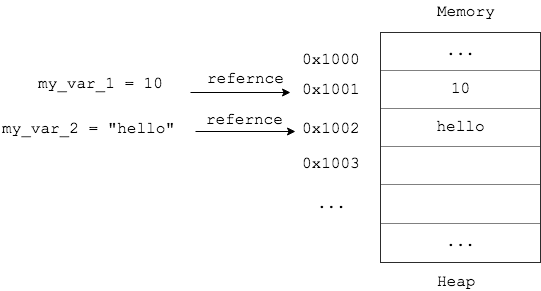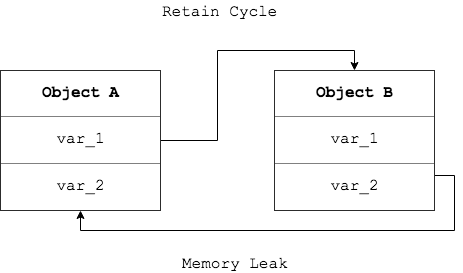# Variables and Memory Part 1

### Memory Model``````a = 10
print(hex(id(a))) #0x107e2bb90
``````

### Reference Count

``````my_var = 10
other_var = my_var
``````

``````import sys
a = [1,2,3]
sys.getrefcount(a) #2
``````

``````import ctypes

ref_count(id(a)) #1
``````

``````a_address = id(a)
a = None
``````

### Garbage Collection``````import ctypes
import gc

def object_by_id(object_id):
for  obj in gc.get_objects():
if id(obj) == object_id:
return "Object Exists"
``````

``````class A:
def __init__(self):
self.b = B(self)
print(f'A: self:{hex(id(self))},b:{hex(id(self.b))}')

class B:
def __init__(self, a):
self.a = a
print(f'B: self:{hex(id(self))},a:{hex(id(self.a))}')

gc.disable()
a = A()

# B: self:0x10aa2b208,a:0x10aa2b160
# A: self:0x10aa2b160,b:0x10aa2b208
``````

``````id_a = id(a)
id_b = id(a.b)
print(ref_count(id_a)) #2
print(ref_count(id_b)) #1
print(object_by_id(id_a)) #Object Exists
print(object_by_id(id_b)) #Object Exists
``````

`a`的引用计数为2，`a`被自身引用和被`b.a`引用，`b`的引用计数为1，只被`a.b`引用，符合推理，同样，`a,b`均存在与GC的列表中。接下来我们将`a`的引用计数-1

``````a = None
print(ref_count(id_a)) #1
print(ref_count(id_b)) #1
``````

``````gc.collect()
print(ref_count(id_a)) #0
print(ref_count(id_b)) #0
``````

### Important Note

• `Jython`可将python代码编译为JVM可识别的某种中间代码(类似`javac`)从可使其运行在JVM中
• `IronPython`是用`C#`编写的，可以运行在`.net`平台上的Python引擎
• `PyPy`是用Python写的一个Python解释器

（全文完）